Exponents 4
Set 4 (10 Questions)
Determine the prime factors of all numbers through 50 and write the numbers as the product of their prime factors by using exponents to show multiples of a factor (e.g., 24 = 2 x 2 x 2 x 3 = two cubed times three)
From Mr. Anker Tests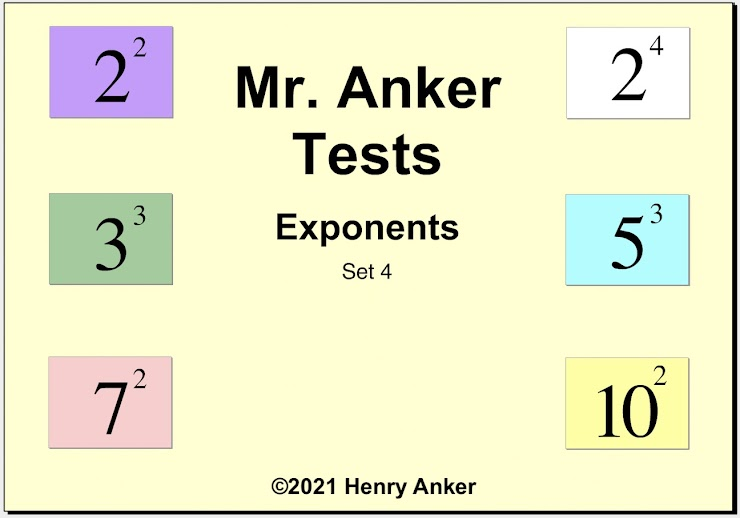Exponents Help Slide 1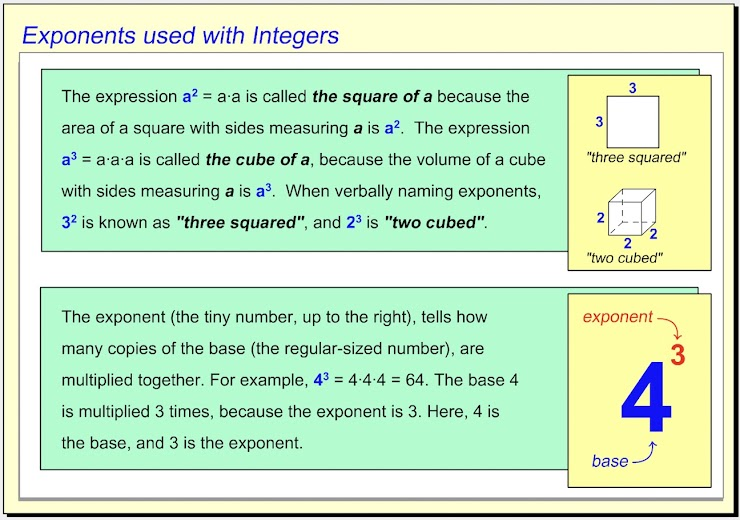Exponents Help Slide 2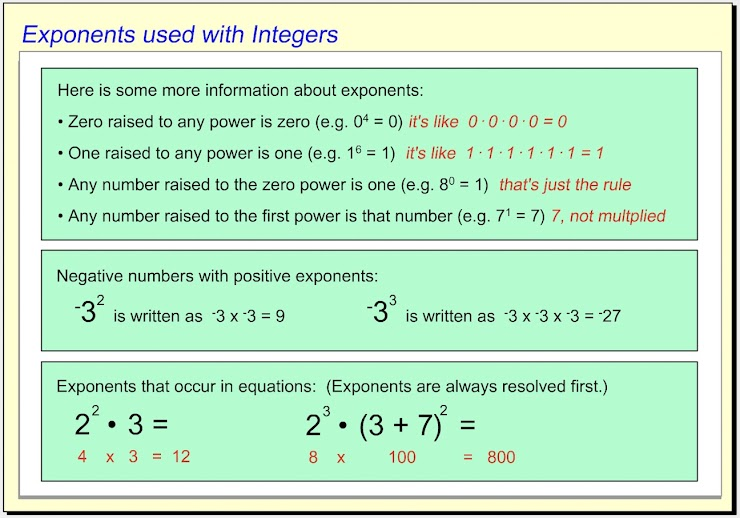Exponents Help Slide 3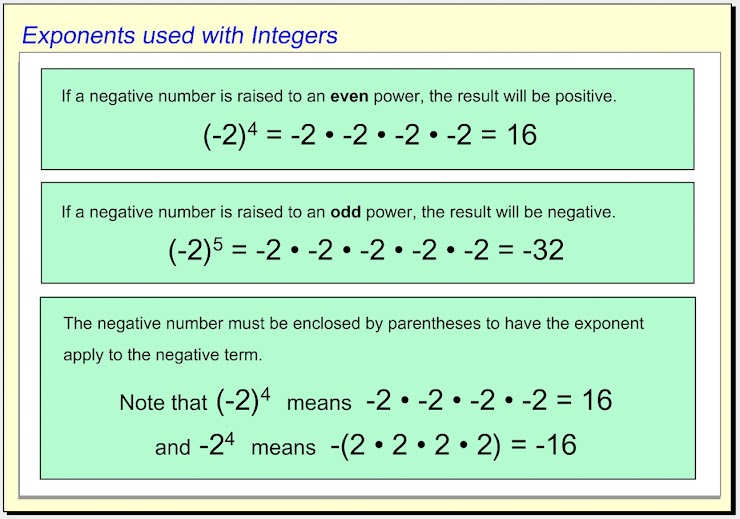1.  Which of the choices below is equal to the integer with the exponent shown? *
1 point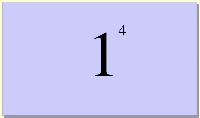2.  Use the dropdown menu to make this statement true:  "Six squared is __________ the product of two times three squared." *
1 point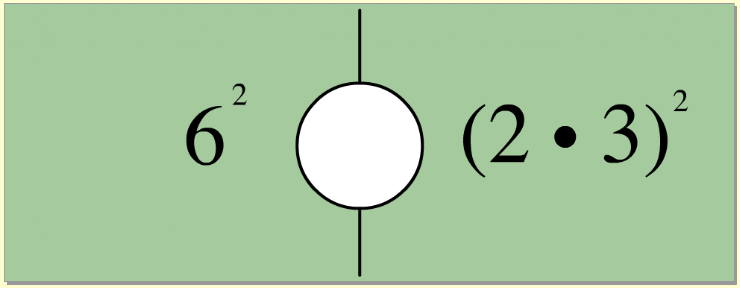3.  Which of the choices below is equal to the integer with the exponent shown? *
1 point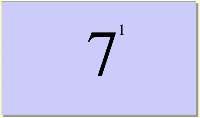4.  Use the dropdown menu to make this statement true:  "Seven squared is_________ the sum of five and two squared." *
1 point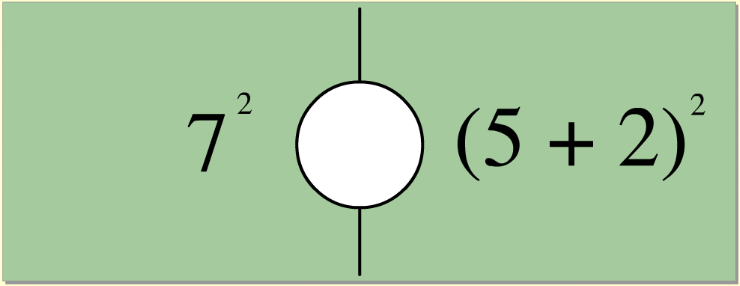5.  Which choice below is the correct way to name the integer with the exponent shown? *
1 point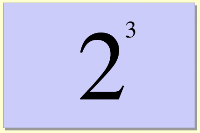6. Use the dropdown menu to make this statement true:  "The difference of nine minus six cubed is ___________ the sum of four and one squared." *
1 point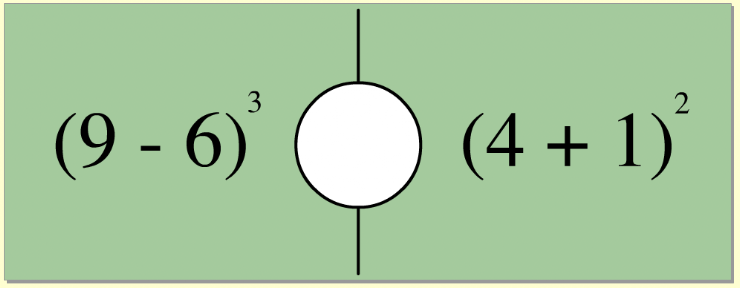7.  On the line below, enter the equal value as a whole number. *
1 point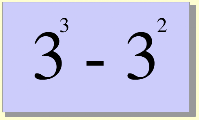8. Use the dropdown menu to make this statement true:  "Nine to the first power is ___________ three squared." *
1 point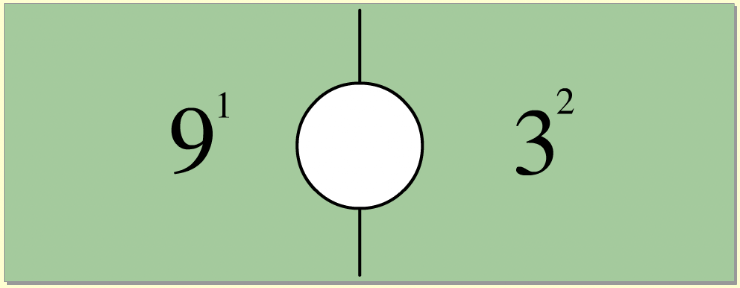9.  Which of the choices below is equal to the integer with the exponent shown? *
1 point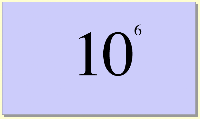10.   On the line below, enter the equal value as a whole number. *
1 point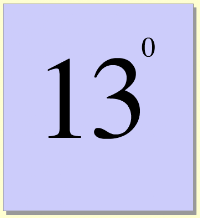Submit
Clear form